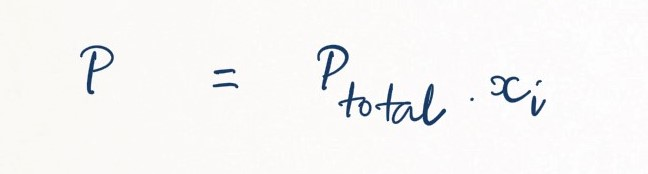Complimentary 1-hour tutoring consultation

MCAT Content / Gas Phase / Partial Pressure Mole Fraction

### Partial Pressure, Mole Fraction

Topic: Gas Phase

The partial pressure of an individual gas is equal to the total pressure multiplied by the mole fraction of that gas.

Because it is dependent solely on the number of particles and not the identity of the gas, the Ideal Gas Equation applies just as well to mixtures of gases as it does to pure gases. Dalton correctly reasoned that the low density and high compressibility of gases were indicative of the fact that they consisted mostly of empty space; from this, it Dalton concluded that when two or more different gases occupy the same volume, they behave entirely independently of one another. Dalton’s Law (also called Dalton’s Law of Partial Pressures) states that the total pressure exerted by the mixture of non-reactive gases is equal to the sum of the partial pressures of individual gases.

The mole fraction is a way of expressing the relative proportion of one particular gas within a mixture of gases. We do this by dividing the number of moles of a particular gas i by the total number of moles in the mixture:The partial pressure of one individual gas within the overall mixtures, pi, can be expressed as follows:where xi is the mole fraction.

We know from Boyle’s Law that the total pressure of the mixture depends solely on the number of moles of gas, regardless of the types and amounts of gases in the mixture. The Ideal Gas Law reveals that the pressure exerted by a mole of molecules does not depend on the identity of those particular molecules. Dalton’s Law allows us to calculate the total pressure in a system when we know each gas individual contribution.

Pressure can also change with height. If the density of the gas does not change, changes in height can lead to changes in pressure.

This can be related to by the equation Pressure = density x gravitational acceleration x height. This will give the pressure of a gas at different atmospheric heights.

Practice Questions

MCAT Official Prep (AAMC)

Physics Online Flashcards Question 2

Practice Exam 3 C/P Section Question 58

Chemistry Question Pack Question 94

Key Points

• The partial pressure of an individual gas is equal to the total pressure multiplied by the mole fraction of that gas.

• Boyle’s Law and the Ideal Gas Law tell us the total pressure of a mixture depends solely on the number of moles of gas, and not the kinds of molecules; Dalton’s Law allows us to calculate the total pressure in a system from each gas’ individual contribution.

Key Terms

Mole fraction: number of moles of one particular gas divided by the total moles of gas in the mixture

Dalton’s Law of Partial Pressures: the total pressure exerted by the mixture of non-reactive gases is equal to the sum of the partial pressures of each individual gas; also known as Dalton’s Law of Partial Pressures

Billing Information